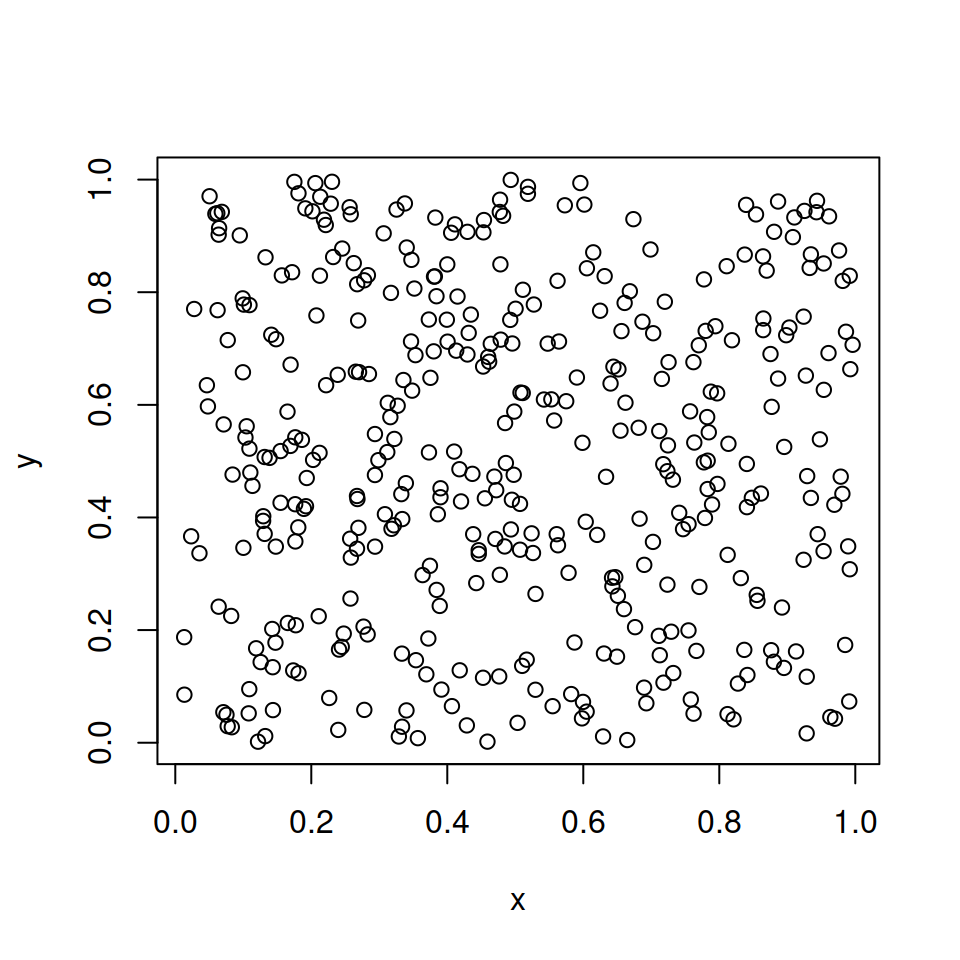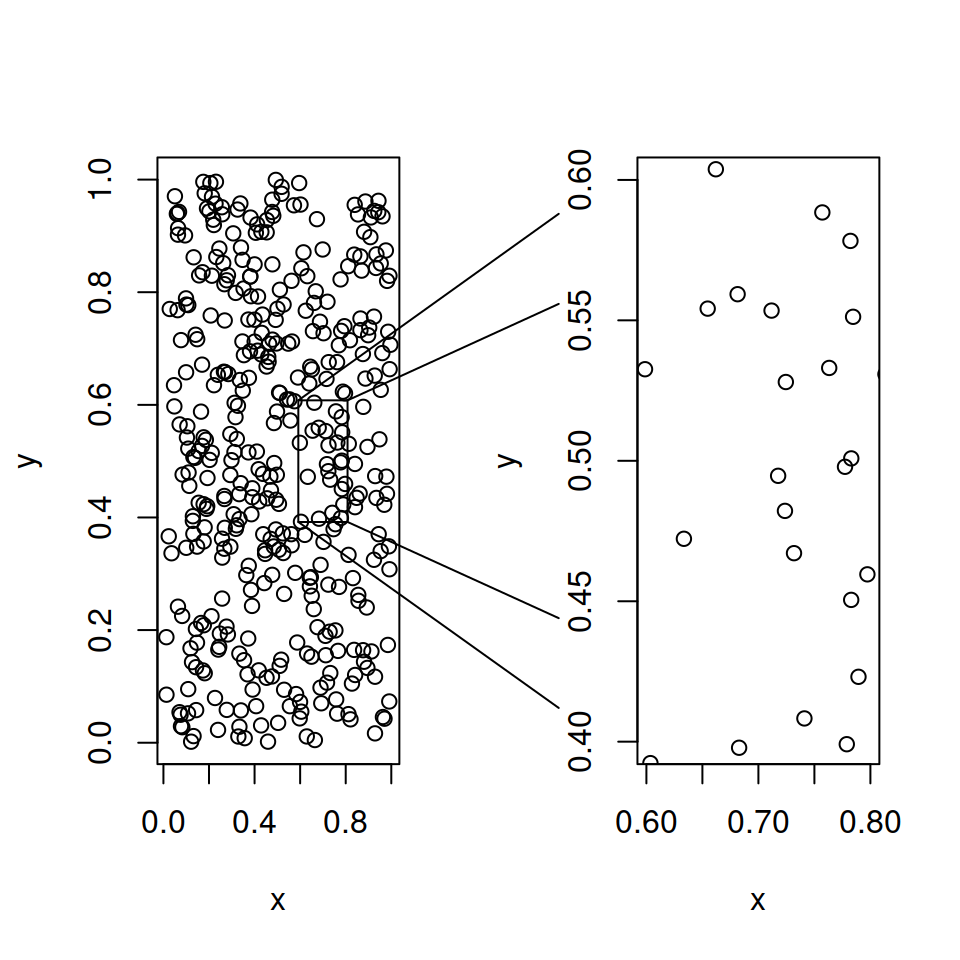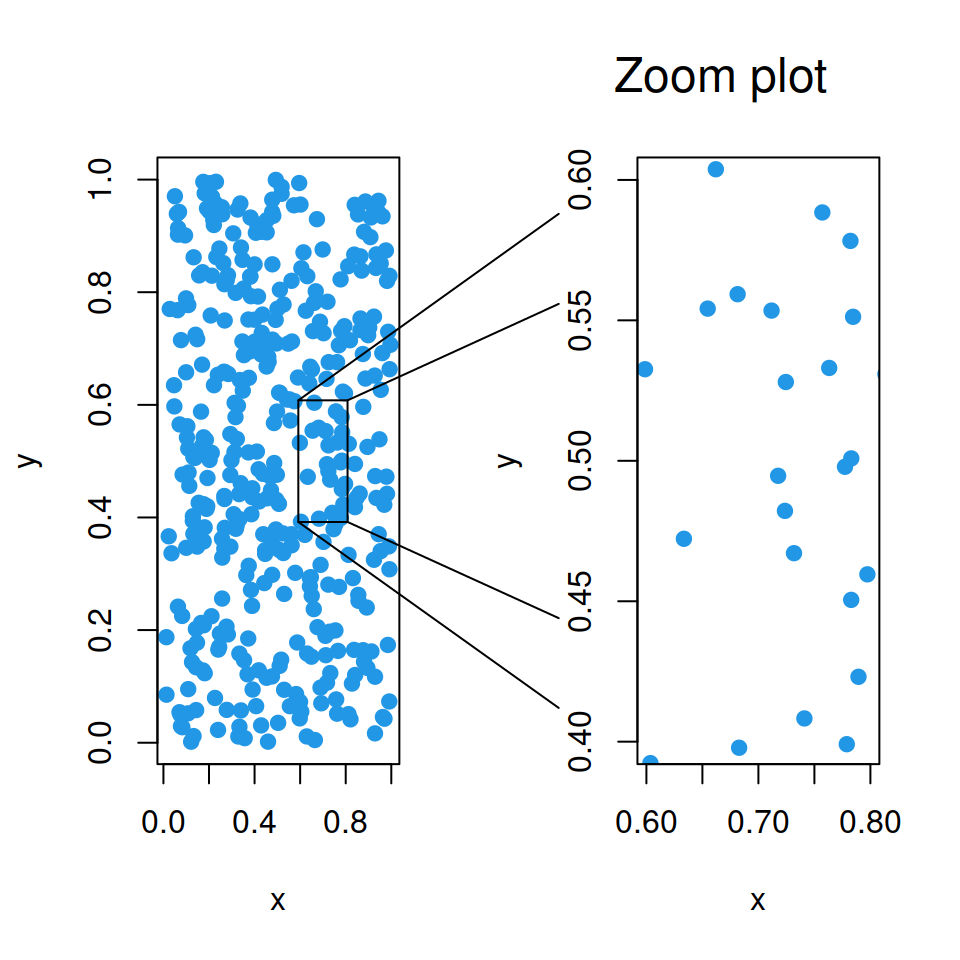# Zoom out of plot in R

plotrix

Jim Lemon

## Zoom in

Given two vectors of the same length it is possible to create a scatter plot with the `plot` function as in the example below.

``````# Data
set.seed(1)
x <- runif(400)
y <- runif(400)

# Scatter plot
plot(x, y)``````If you want to zoom in an specific area you can make use of the `zoomInPlot` function from `plotrix` package. This function will highlight the corresponding area in the chart and create a new plot out of the first with the zoomed data. You just need to specify the X-axis and Y-axis limits for the expanded plot with `rxlim` and `rylim`, respectively.

``````# install.packages("plotrix")
library(plotrix)

# Data
set.seed(1)
x <- runif(400)
y <- runif(400)

# Scatter plot with zoom
zoomInPlot(x, y,                # Data
rxlim = c(0.6, 0.8), # X-axis limits
rylim = c(0.4, 0.6)) # Y-axis limits``````Note that the proportions of the plots depend on the device size. If you increase the size of your plot or if you modify the margins it is possible to get squared plots as in the first figure.

### Color customization

You can also change the symbol of the plots, its color and specify other additional arguments of the `plot` function inside `zoomInPlot`. In addition, there is an argument named `zoomtitle` which can be used to set a title for the zoomed plot.``````# install.packages("plotrix")
library(plotrix)

# Data
set.seed(1)
x <- runif(400)
y <- runif(400)

# Scatter plot with zoom
zoomInPlot(x, y,                    # Data
pch = 19,                # Symbol
col = 4,                 # Color
zoomtitle = "Zoom plot", # Title
rxlim = c(0.6, 0.8),
rylim = c(0.4, 0.6))``````